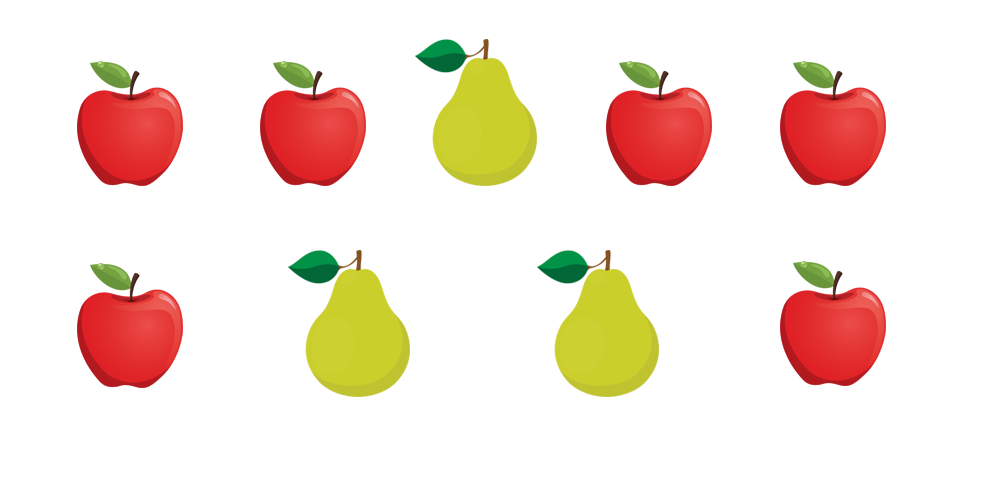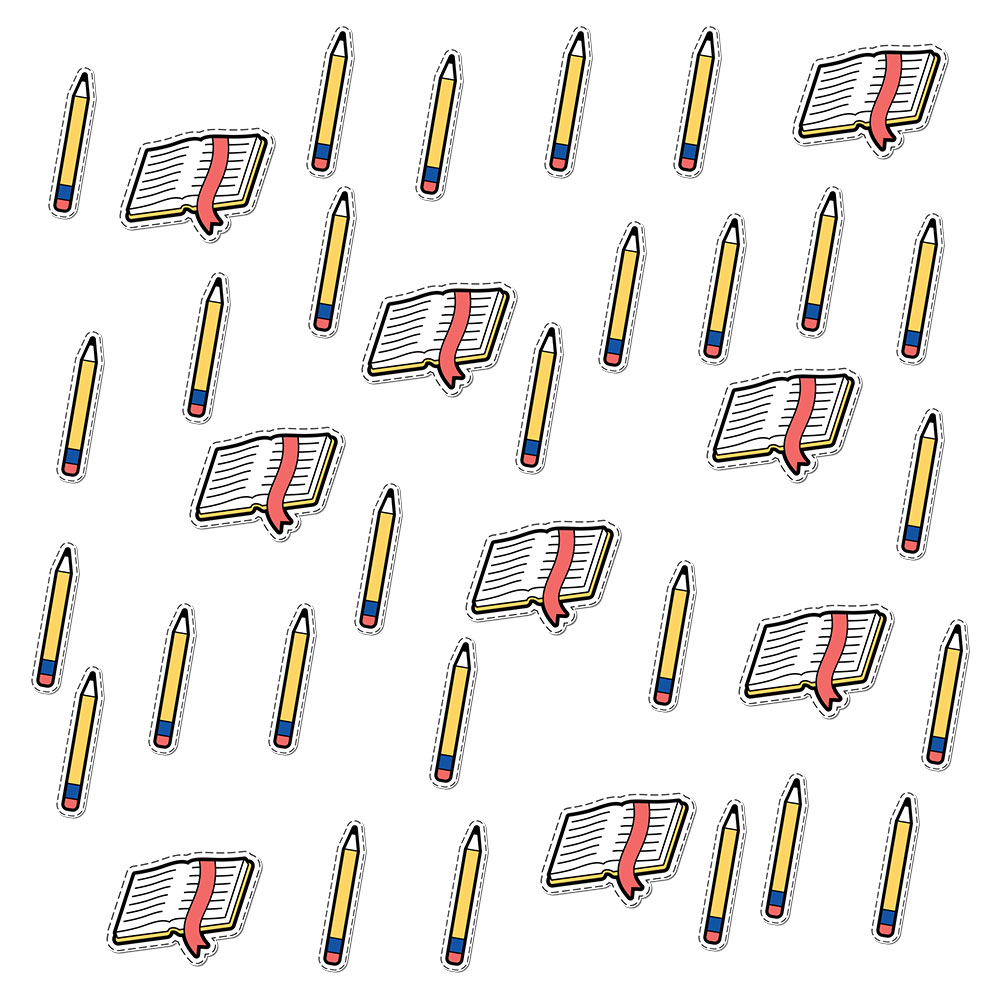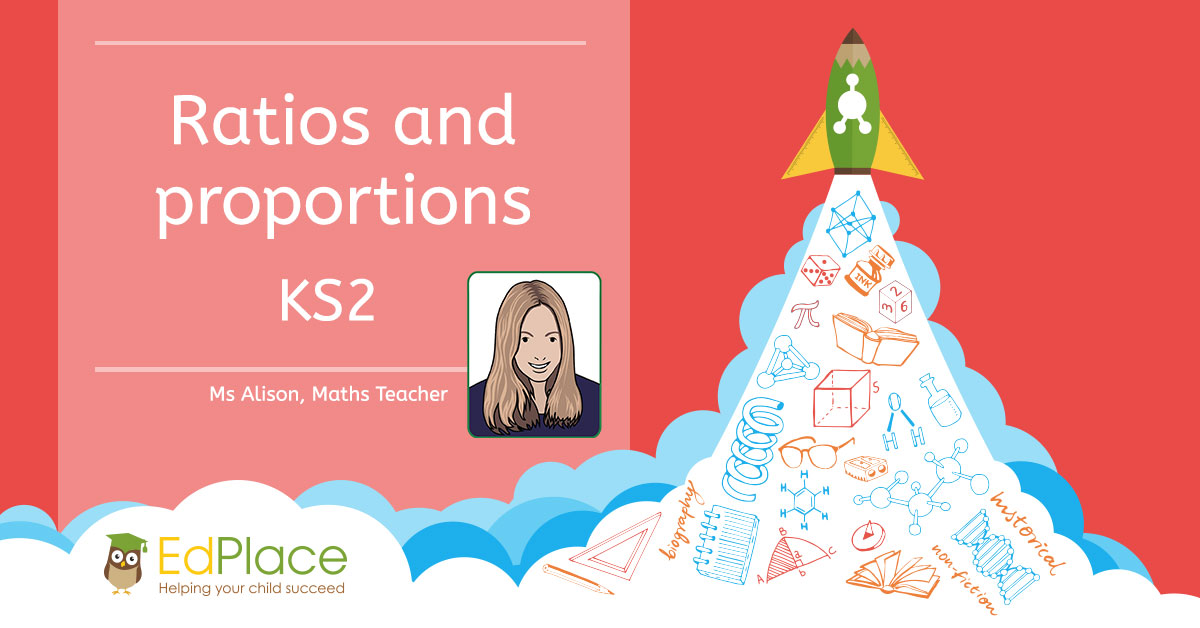# Learning ratios and proportions in KS2

Ratio and proportion are now an important part of the KS2 maths curriculum. This may seem daunting to those of you who are nervous about maths, but it's not scary...honest!  In fact, once you get the hang of it, ratio and proportion can even be fun! Let's get into it.

# Ratios

Ratios are simply a way to compare numbers or quantities. They tell us how much there is of one thing compared to another. We use the : symbol to separate the numbers being compared. Look at the example below;Let's compare the number of apples to the number of pears.

There are 9 pieces of fruit; 6 apples and 3 pears.

For every two apples, there is one pear. This means the ratio is 2:1.

But what happens if we have bigger numbers...?Toby’s class appears to have a lot more pencils than books...but what is the ratio of boys to girls?

There are 36 pencils and books altogether, 27 pencils and 9 books.

We can write this as 27:9.

To make it easier to understand, we might want to simplify the ratio in the same way that we might simplify a fraction. 27 and 9 are both in the 9 x table. This is the highest common factor. Therefore, 27 ÷ 9 = 3 and 9 ÷ 9 = 1. The simplified ratio is 3:1 (or 3 boys for every girl).

# Proportion

Proportion tells us how many of one thing there is out of the whole number. Let's take another look at the examples above, the proportions look like this;

The proportion of apples is 6 out of 9.

The proportion of pears is 3 out of 9.

The proportion of boys is 27 out of 36.

The proportion of pears is 9 out of 36.

Proportion can also be represented as a decimal, fraction or percentage, as shown below;

 Decimal Fraction Percentage The proportion of apples is 6 out of 9 6 ÷ 9 = 0.66 6/9 or 2/3 66% The proportion of pears is 3 out of 9 3 ÷ 9 = 0.33 3/9 or 1/3 33% The proportion of boys is 27 out of 36 27 ÷ 36 = 0.75 27/36  or 3/4 75% The proportion of pears is 9 out of 36 9 ÷ 36 = 0.25 9/36 or 1/4 25%

# Solving ratio and proportion questions

Often, you'll be given information and will have to use your knowledge of ratio, proportion, percentages, decimals or fractions to work out the missing facts. It’s important, therefore, that you have a secure understanding of how these skills work together.

Here are some sample SAT-style questions and answers to give you an idea of what you might be asked in an exam;

## Question 1

Charlie and Jade have some sweets. Altogether, they have 14 sweets, and Charlie has 2 more than Jade.

How many sweets do Charlie and Jade have each?

What proportion of the sweets does Charlie have?

## Question 2

Raisins cost 60p for 100 grams.

What is the cost of 350 grams of raisins?

Answer: 60p x 3 = £1.80 + 30p – ½ of 60 = £2.10

## Question 3

Two letters have a total weight of 120 grams

One letter weighs twice as much as the other.

Write the weight of the heavier letter.

Answer: 120 divided by 3 x 2 = 80g

# Worksheets and Practice

Learning ratios and proportions in KS2 can be really good fun and once mastered, can be useful for all sorts of comparisons. We at EdPlace are here to help and support you with your learning. We have plenty of great worksheets to teach you about using ratios and proportions. We’ve listed a few of the most relevant here, but please do browse through our website or search for ‘ratio’ or ‘proportion’ in order to find many, many more resources.

Year 5 – Equal or not equal 12

Year 6 – Ratio questions 1

Year 6 – Unequal sharing using multiples

Year 6 – Unequal sharing using fractions

Year 6 – Similar shape problems

Year 6 – Solving scale drawing problems

Year 6 – Mental calculations 12

https://www.edplace.com/worksheet_info/maths/keystage2/year6/topic/930/4479/mental-calculations-1

Year 6 - Ratio and proportion: reading a pie chart 1, 2

Year 6 - Ratio and proportion: reading a pie chart 2

# Further Information

If you enjoy learning ratios and proportions and want to give yourself a challenge, why not try some of the games on the BBC website or do some puzzles and problems set by the NRich team from the University of Cambridge?

https://www.bbc.co.uk/education/topics/zsq7hyc

https://nrich.maths.org/9003###### AUTHOR, MS ALISON – MATHS TEACHER.